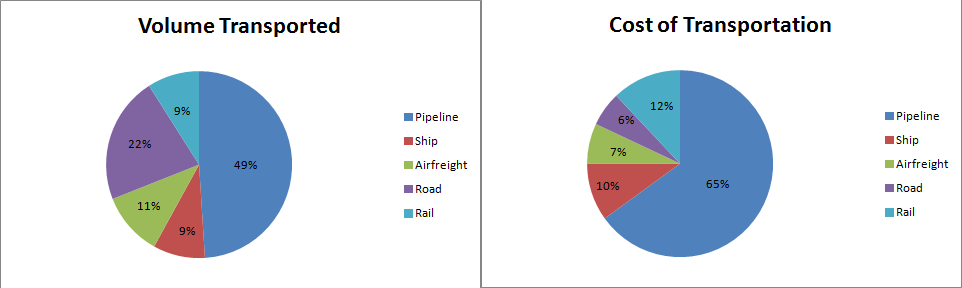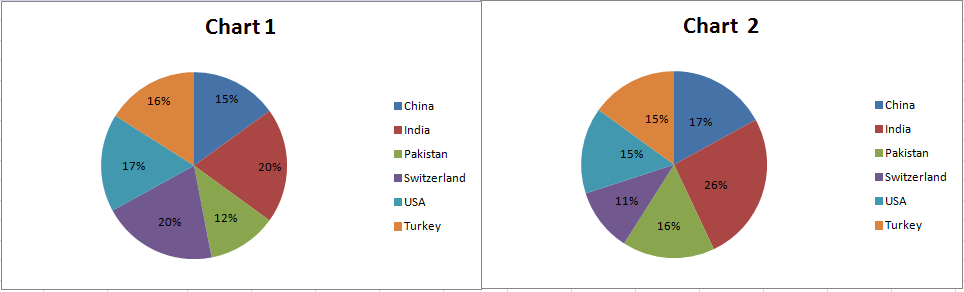# Pie Chart Questions for CAT

1
5927

In recent CAT papers, the combinations of pie charts data with other forms are appearing more rather than only pie charts. But solving this pie charts questions, will help you to improve your speed while solving such questions.

Pie Chart Questions for CAT:

More Questions for Practice on DI-Pie charts

Instructions: (1 to 3)

For the following three questions: Answer the questions based on the pie charts given below.

Chart 1 shows the distribution of 12 million tonnes of crude oil transported through different modes over a specific period of time.

Chart 2 shows the distribution of the cost of transporting this crude oil. The total cost was Rs. 30 million.Question 1:

The cost in rupees per tonne of oil moved by rail and road happens to be roughly

a) Rs. 3
b) Rs. 1.5
c) Rs. 4.5
d) Rs. 8

Question 2:

From the charts given, it appears that the cheapest mode of transport is

b) rail
c) pipeline
d) ship

Question 3:

If the costs per tonne of transport by ship, air and road are represented by P, Q and R respectively, which of the following is true?

a)R > Q > P
b)P > R > Q
c)P > Q > R
d)R > P > Q

Solutions: (1 to 3)

Total tonnes of transportation by both rail and road is about  31*12/100 = 3.72 million tonnes and total cost incured 18*30/100 = 5.4 .
Hence required value is 5.4/3.72 which is about 1.5.
Hence option B .

Cheapest mode of transport will be the one which will have highest transport volume and comparatively lowest cost. We can figure out from the graph that Road have wide gap with very less costs.

For Rail, Cost = 12/9
For Pipeline, = 65/49
For Ship, Cost = 10/9

If the costs per tonne of transport by ship, air and road are represented by P, Q and R respectively.

P = 10/9
Q = 7/11
R = 6/22
We can see that P>Q and Q>R  and P>R. Hence option C.

Instructions: 4 to 5

Chart 1 shows the distribution by value of top 6 suppliers of MFA Textiles in 1995.

Chart 2 shows the distribution by quantity of top 6 suppliers of MFA Textiles in 1995.

The total value is 5760 million Euro (European currency). The total quantity is 1.055 million tonnes.Question 4:

The country which has the highest average price is

a) USA
b) Switzerland
c) Turkey
d) India

Question 5:

The average price in Euro per kilogram for Turkey is roughly

a) 6.20
b) 5.60
c) 4.20
d) 4.80

Solutions: (4 to 5)

The country which has the highest average price would have low quality and high value when compared to other countries..

Switzerland fits the above description.

Hence, option B is the answer.